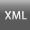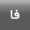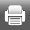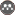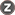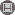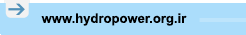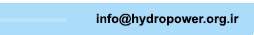[Home ] [Archive]   [ فارسی ]Volume 6, Issue 22 (12-2019)2019, 6(22): 1-12 Back to browse issues page
Predicting discharge coefficient of Triangular Plan Form Weirs using Hybrid Model based on Fuzzy Systems and Differential Evolution Algorithm
Kermanshah Branch, Islamic Azad University , byaghubi@gmail.com
Abstract:   (498 Views)
Weirs are used to adjust and measure the flow within open conduit. Also, weirs are applied in various shapes like rectangular, triangular, circular and triangular plan forms. In this study, the discharge coefficient of triangular plan form weirs was predicted using a hybrid model based on fuzzy systems (ANFIS) and differential evolution (DE) algorithm. Adaptive neuro Also, to examine the performance of hybrid models, the Monte Carlo simulations (MCs) were used. In this research, the k-fold Cross Validation with k equal to 5 was used for evaluation of the models ability. Then, the six hybrid models were defined using input parameters. According to simulation results, the superior model predicted the discharge coefficient in terms of Froude number, weir vertex angle, the ratio of weir length to weir height, the ratio of head over the crest of the weir to weir height and channel width to weir length. For superior model, the Mean Absolute Percentage Error (MAPE), Root Mean Square Error (RMSE) and correlation coefficient (R) were calculated equal to 1.644, 0.016 and 0.972, respectively. Also, the Froude number was identified as the most effective parameter to model the discharge coefficient of triangular plan form weirs
Keywords: Adaptive Neuro-Fuzzy Inference Systems, Differential evolution algorithm, Discharge coefficient, Triangular plan form weir
Type of Study: Research | Subject: سد و سازه
Send email to the article author